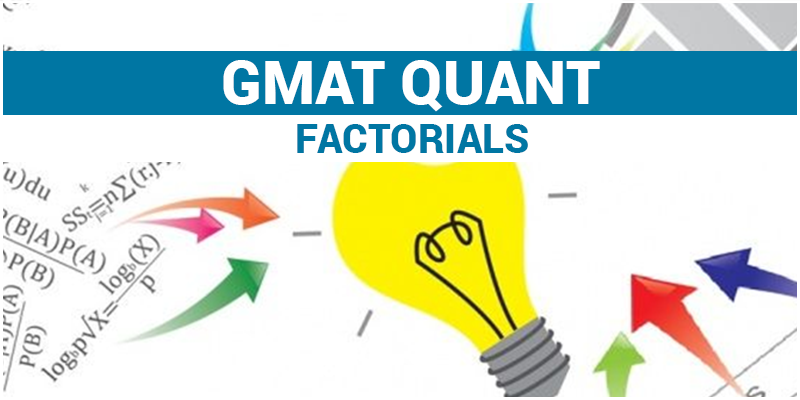# GMAT Quant: FactorialsFactorial representation is given as

N! = N.(N-1).(N-2).(N-3)……..3.2.1

The general usage for factorial lies majorly in permutation and combination. However, there might be few questions in GMAT that will be related to numbers. Don’t get frightened !!. this is just a way to represent the product of consecutive integer.

Let’s look at some problems:

1. What maximum power of 10 will divide the number 30!?

In 30! If we have to count the power of 10, we need to know the maximum power of 5 and maximum power of 2. Since this number will have abundant power of 2 i.e even numbers as compared to number of 5, we will be counting the number of 5’s.

30! = 30.29……25……20……15…….10….5……3.2.1

Number of 5’s in 30.25.20.15.10.5 = 7.

Hence maximum power of 10 that can divide 30! Will be 7.

1.  What is the maximum power of 2 in the expression 10! + 11! +12!…………20!?

10! + 11! +12!…………20!

10! (1 + 11 +12.11 + 13.12.11 +………..+ 20.19.18……13.12.11)

10! (12 +12.11 + 13.12.11 +………..+ 20.19.18……13.12.11)

10!.12 (1 +11 +13.11+14.13.11+…………..+20.19.18……..13.11)

10!.12 (12 +13.11+14.13.11+…………..+20.19.18……..13.11)

We can see in the bracket that every term is even except 13.11 which is odd

We know even + even is even and even + odd is odd

Therefore bracket will yield an odd number.

So all the power to 2 will be there in 10!. 12

Number of power of 2 in 10! = [10/2] + [10/4] +{10/8] = 8, where [] denotes the greatest integer.

Number of power of 2 in 12 is 2

Hence total number of power of 2 in the expression is 8 +2 =10